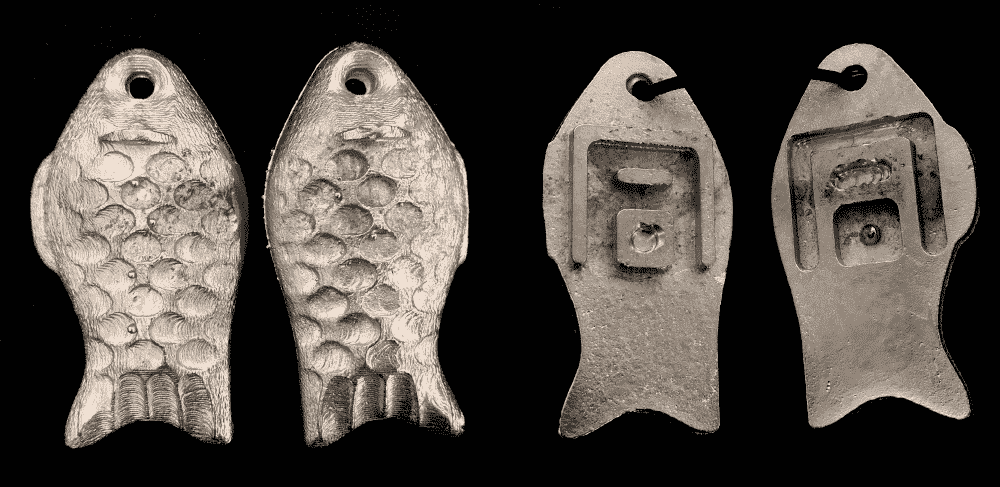# Molding and Casting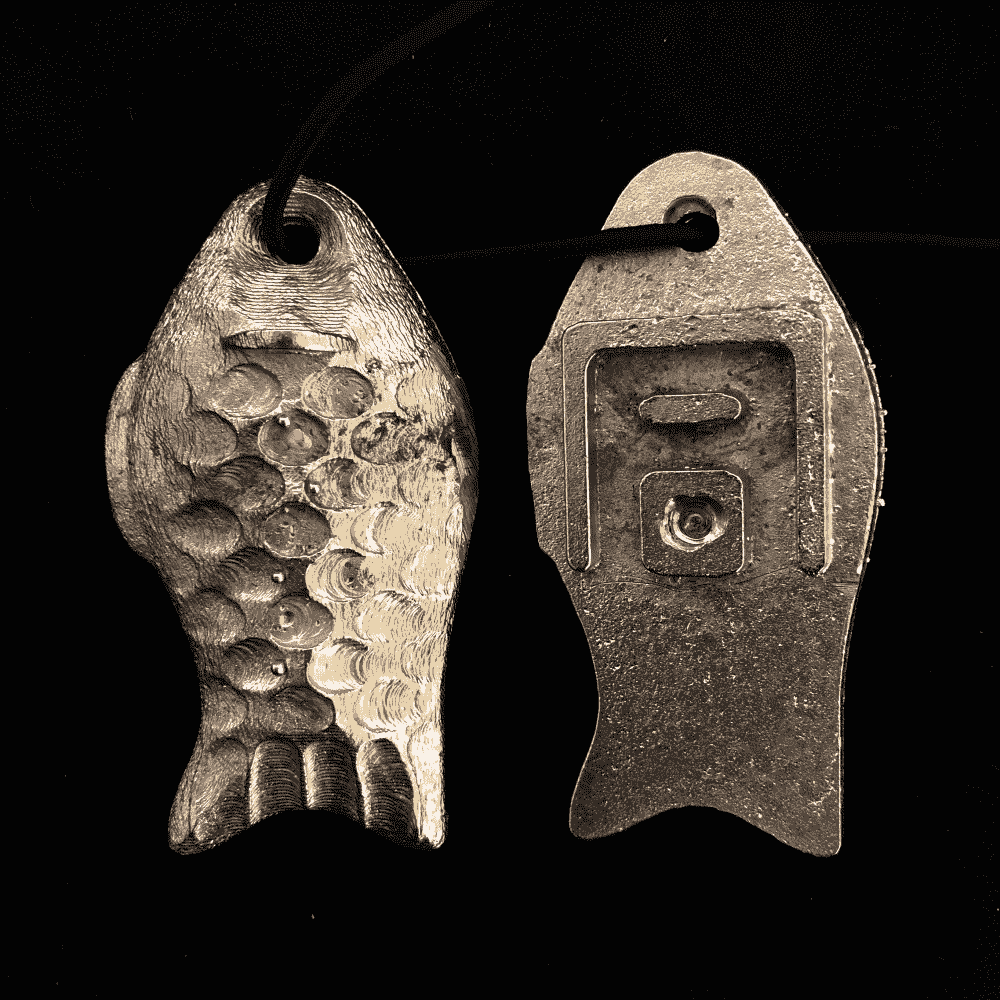This week we're making molds and casting parts from them.

Given the small size of the materials we were provided with, initially I wanted to make a Chinese seal. However, since I've already hand-carved seals before, and that Cathy from our section also expressed interest in making a seal, I decided to look for another idea. I also realized that since the endmill we were using (1/8 inch) was quite fat, either the seal would have to be quite big (and not very intricate-looking), or it would not be able to fit adequate number of characters on it.

So I decided to make a "Fu", or “commander's tally”, as it had been roughly translated. These are usually a set of two pieces, which have positive (relief) and negative (engraved) versions of an identical pattern, that allow them to fit into each other. They were used in ancient China as tokens for authentication. Each party holds one of the pieces, and when exchanging confidential information, they place the pieces together: if they fit, the integrity of the message is verified, and vice versa. The most common use case was for passing military commands from the monarch to the marshal, but they were also used by high officials as “passports” for entering the palace.

These tallies came in a variety of shapes, most commonly in that of a tiger, but fish and turtle shaped ones were also used. Here's a photo of a Tang Dynasty fish Fu on which my design is based. On it you can see the character “同” (meaning sameness, equivalence) used as the interlocking pattern.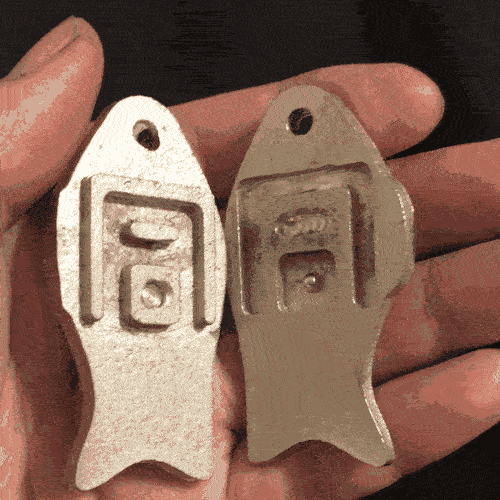## Design

Again I used my JAD (JavaScript-Aided-Design) skills to produce the 3D model. However, unlike how I built the mesh directly for my 3D printing project, this time I first generated a 2D depthmap, which was then offset and converted to STL for machining. There were a couple of benefits:

• Unmachinable design (due to overhang/undercut) becomes impossible to generate, since z is a function of (x,y).
• 2D images are easier to manipulate (and view) than 3D models.
• mods by prof. Neil seems to also involve depthmaps for computing 3D toolpaths, so it's likely not a bad idea.

Since I was planning to make a two-part mold of two pieces, the design would appear to have 4 shapes.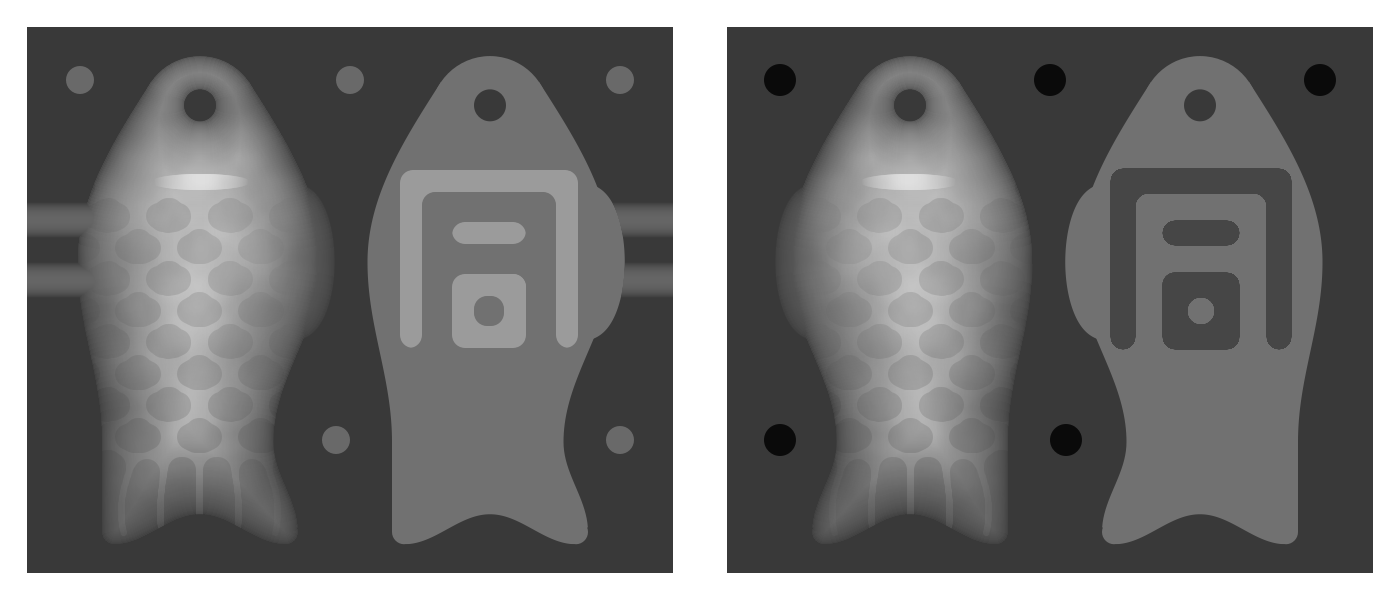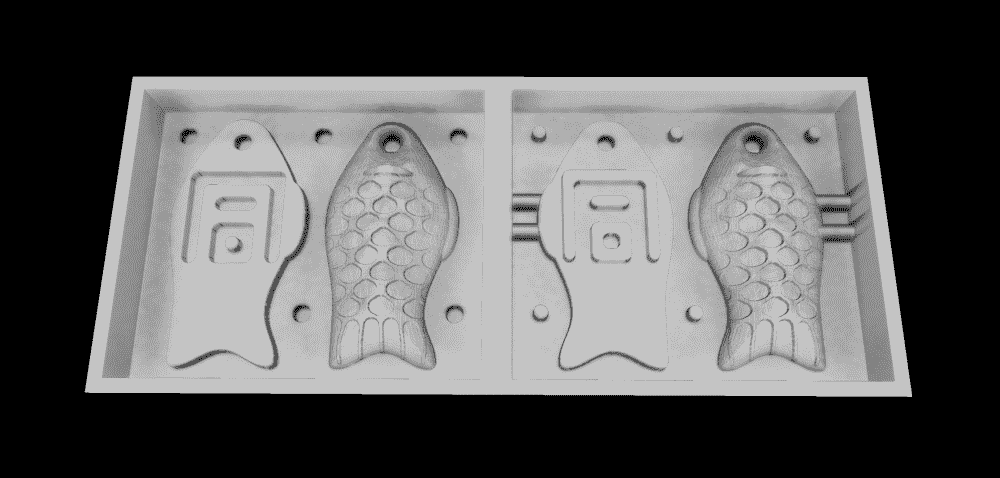You can find the full code below, or try the online demo:

const PI = Math.PI;
const cos = Math.cos;
const sin = Math.sin;

const W = 1400; // unit: 1/200 inch
const H = 600;

var tool_d = 26;
var body_h = 450;
var body_w = 245;
var tong_x = -118;
var tong_y = 135;
var reg_r = 14;
var reg_p = 80;
var tube_w = 40;
var tube_l = 100;
var tube0_y = 200;
var tube1_y = 260;
var scale_n = 5;
var hole_r = 16;

const tong = \

dMMMMMb
M'   "M
M <M> M
M     M
M dMb M
M MOM M
v qMp v
.split('\n');

const is_node = typeof process != 'undefined';

let fs;
let createCanvas;
let findContours,approxPolyDP;

if (is_node){
fs = require('fs');
;({createCanvas} = require('node-canvas'));
;({findContours,approxPolyDP} = require('./findcontours.js'));
}else{
createCanvas = function(w,h){
let cnv = document.createElement('canvas');
cnv.width = w;
cnv.height = h;
return cnv;
}
;({findContours,approxPolyDP} = FindContours);
}

var cnv = createCanvas(W,H);
let ctx = cnv.getContext('2d');

let jsr = 0x5EED;
function rand(){
jsr^=(jsr<<17);
jsr^=(jsr>>13);
jsr^=(jsr<<5);
return (jsr>>>0)/4294967295;
}

let PERLIN_YWRAPB = 4; let PERLIN_YWRAP = 1<<PERLIN_YWRAPB;
let PERLIN_ZWRAPB = 8; let PERLIN_ZWRAP = 1<<PERLIN_ZWRAPB;
let PERLIN_SIZE = 4095;
let perlin_octaves = 4;let perlin_amp_falloff = 0.5;
let scaled_cosine = function(i) {return 0.5*(1.0-Math.cos(i*PI));};
let perlin;
let noise = function(x,y,z) {
y = y || 0; z = z || 0;
if (perlin == null) {
perlin = new Array(PERLIN_SIZE + 1);
for (var i = 0; i < PERLIN_SIZE + 1; i++) {
perlin[i] = rand();
}
}
if (x<0) { x=-x; } if (y<0) { y=-y; } if (z<0) { z=-z; }
var xi=Math.floor(x), yi=Math.floor(y), zi=Math.floor(z);
var xf = x - xi; var yf = y - yi; var zf = z - zi;
var rxf, ryf;
var r=0; var ampl=0.5;
var n1,n2,n3;
for (var o=0; o<perlin_octaves; o++) {
var of=xi+(yi<<PERLIN_YWRAPB)+(zi<<PERLIN_ZWRAPB);
rxf = scaled_cosine(xf); ryf = scaled_cosine(yf);
n1  = perlin[of&PERLIN_SIZE];
n1 += rxf*(perlin[(of+1)&PERLIN_SIZE]-n1);
n2  = perlin[(of+PERLIN_YWRAP)&PERLIN_SIZE];
n2 += rxf*(perlin[(of+PERLIN_YWRAP+1)&PERLIN_SIZE]-n2);
n1 += ryf*(n2-n1);
of += PERLIN_ZWRAP;
n2  = perlin[of&PERLIN_SIZE];
n2 += rxf*(perlin[(of+1)&PERLIN_SIZE]-n2);
n3  = perlin[(of+PERLIN_YWRAP)&PERLIN_SIZE];
n3 += rxf*(perlin[(of+PERLIN_YWRAP+1)&PERLIN_SIZE]-n3);
n2 += ryf*(n3-n2);
n1 += scaled_cosine(zf)*(n2-n1);
r += n1*ampl;
ampl *= perlin_amp_falloff;
xi<<=1; xf*=2; yi<<=1; yf*=2; zi<<=1; zf*=2;
if (xf>=1.0) { xi++; xf--; }
if (yf>=1.0) { yi++; yf--; }
if (zf>=1.0) { zi++; zf--; }
}
return r;
};

function ellipse(ctx,x,y,rx,ry){
ctx.beginPath();
ctx.ellipse(x,y,rx,ry,0,0,PI*2);
ctx.fill();
}

function seg_isect(p0x, p0y, p1x, p1y, q0x, q0y, q1x, q1y, is_ray = false) {
let d0x = p1x - p0x;
let d0y = p1y - p0y;
let d1x = q1x - q0x;
let d1y = q1y - q0y;
let vc = d0x * d1y - d0y * d1x;
if (vc == 0) {
return null;
}
let vcn = vc * vc;
let q0x_p0x = q0x - p0x;
let q0y_p0y = q0y - p0y;
let vc_vcn = vc / vcn;
let t = (q0x_p0x * d1y - q0y_p0y * d1x) * vc_vcn;
let s = (q0x_p0x * d0y - q0y_p0y * d0x) * vc_vcn;
if (0 <= t && (is_ray || t < 1) && 0 <= s && s < 1) {
let ret = {t, s, side: null, other: null, xy: null};
ret.xy = [p1x * t + p0x * (1 - t), p1y * t + p0y * (1 - t)];
ret.side = pt_in_pl(p0x, p0y, p1x, p1y, q0x, q0y) < 0 ? 1 : -1;
return ret;
}
return null;
}
function pt_in_pl(x, y, x0, y0, x1, y1) {
let dx = x1 - x0;
let dy = y1 - y0;
let e = (x - x0) * dy - (y - y0) * dx;
return e;
}

function pt_in_poly(p,poly){
let n = 0;
let q = [p+Math.PI, p+Math.E];
for (let i = 0; i < poly.length; i++){
let h = seg_isect(...p,...q,...poly[i],...poly[(i+1)%poly.length],true);
// console.log(h);
if (h){
n++;
}
}
// console.log(p,q,poly,n);
return n % 2 == 1;
}

function isect_circ_line(cx,cy,r,x0,y0,x1,y1){
//https://stackoverflow.com/a/1084899
let dx = x1-x0;
let dy = y1-y0;
let fx = x0-cx;
let fy = y0-cy;
let a = dx*dx+dy*dy;
let b = 2*(fx*dx+fy*dy);
let c = (fx*fx+fy*fy)-r*r;
let discriminant = b*b-4*a*c;
if (discriminant<0){
return null;
}
discriminant = Math.sqrt(discriminant);
let t0 = (-b - discriminant)/(2*a);
if (0 <= t0 && t0 <= 1){
return t0;
}
let t = (-b + discriminant)/(2*a);
if (t > 1 || t < 0){
return null;
}
return t;
}

function resample(polyline,step){
if (polyline.length <= 2){
return polyline.slice();
}
polyline = polyline.slice();
let out = [polyline.slice()];
let next = null;
let i = 0;
while(i < polyline.length-1){
let a = polyline[i];
let b = polyline[i+1];
let dx = b-a;
let dy = b-a;
let d = Math.sqrt(dx*dx+dy*dy);
if (d == 0){
i++;
continue;
}
let n = ~~(d/step);
let rest = (n*step)/d;
let rpx = a * (1-rest) + b * rest;
let rpy = a * (1-rest) + b * rest;
for (let j = 1; j <= n; j++){
let t = j/n;
let x = a*(1-t) + rpx*t;
let y = a*(1-t) + rpy*t;
let xy = [x,y];
for (let k = 2; k < a.length; k++){
xy.push(a[k]*(1-t) + (a[k] * (1-rest) + b[k] * rest)*t);
}
out.push(xy);
}

next = null;
for (let j = i+2; j < polyline.length; j++){
let b = polyline[j-1];
let c = polyline[j];
if (b == c && b == c){
continue;
}
let t = isect_circ_line(rpx,rpy,step,b,b,c,c);
if (t == null){
continue;
}

let q = [
b*(1-t)+c*t,
b*(1-t)+c*t,
];
for (let k = 2; k < b.length; k++){
q.push(b[k]*(1-t)+c[k]*t);
}
out.push(q);
polyline[j-1] = q;
next = j-1;
break;
}
if (next == null){
break;
}
i = next;

}

if (out.length > 1){
let lx = out[out.length-1];
let ly = out[out.length-1];
let mx = polyline[polyline.length-1];
let my = polyline[polyline.length-1];
let d = Math.sqrt((mx-lx)**2+(my-ly)**2);
if (d < step*0.5){
out.pop();
}
}
out.push(polyline[polyline.length-1].slice());
return out;
}

function dist_transform(b,m,n) {
// Meijster distance
function EDT_f(x, i, g_i) {
return (x - i) * (x - i) + g_i * g_i;
}
function EDT_Sep(i, u, g_i, g_u) {
return Math.floor((u * u - i * i + g_u * g_u - g_i * g_i) / (2 * (u - i)));
}
// First phase
let infinity = m + n;
let g = new Array(m * n).fill(0);
for (let x = 0; x < m; x++) {
if (b[x + 0 * m]){
g[x + 0 * m] = 0;
}else{
g[x + 0 * m] = infinity;
}
// Scan 1
for (let y = 1; y < n; y++) {
if (b[x + y * m]){
g[x + y * m] = 0;
}else{
g[x + y * m] = 1 + g[x + (y - 1) * m];
}
}
// Scan 2
for (let y = n - 2; y >= 0; y--) {
if (g[x + (y + 1) * m] < g[x + y * m]){
g[x + y * m] = 1 + g[x + (y + 1) * m];
}
}
}

// Second phase
let dt = new Array(m * n).fill(0);
let s = new Array(m).fill(0);
let t = new Array(m).fill(0);
let q = 0;
let w;
for (let y = 0; y < n; y++) {
q = 0;
s = 0;
t = 0;

// Scan 3
for (let u = 1; u < m; u++) {
while (q >= 0 && EDT_f(t[q], s[q], g[s[q] + y * m]) > EDT_f(t[q], u, g[u + y * m])){
q--;
}
if (q < 0) {
q = 0;
s = u;
} else {
w = 1 + EDT_Sep(s[q], u, g[s[q] + y * m], g[u + y * m]);
if (w < m) {
q++;
s[q] = u;
t[q] = w;
}
}
}
// Scan 4
for (let u = m - 1; u >= 0; u--) {
let d = EDT_f(u, s[q], g[s[q] + y * m]);

d = Math.floor(Math.sqrt(d));
dt[u + y * m] = d;
if (u == t[q]) q--;
}
}
return dt;
}

function to_3d(){
let faces = [];
let data = ctx.getImageData(0,0,W,H).data;
for (let i = 0; i < H-1; i++){
for (let j = 0; j < W-1; j++){
let x0 = j;
let x1 = j+1;
let y0 = i;
let y1 = i+1;
let z00 = data[(y0*W+x0)*4]/2;
let z10 = data[(y0*W+x1)*4]/2;
let z11 = data[(y1*W+x1)*4]/2;
let z01 = data[(y1*W+x0)*4]/2;
faces.push([[x0,y0,z00],[x1,y0,z10],[x1,y1,z11]]);
faces.push([[x0,y0,z00],[x1,y1,z11],[x0,y1,z01]]);
}
}
return faces;
}

function to_stl_bin(faces){
let nb = 84+faces.length*50;
console.log(writing stl (binary)... estimated ${~~(nb/1048576)} MB); let o = new Uint8Array(nb); let a = new ArrayBuffer(4); let b = new Uint32Array(a); b = faces.length; o.set(new Uint8Array(a),80); for (let i = 0; i < faces.length; i++){ let d = [ faces[i],faces[i],faces[i], faces[i],faces[i],faces[i], faces[i],faces[i],faces[i], ] let a = new ArrayBuffer(36); let b = new Float32Array(a); d.map((x,j)=>b[j]=x); o.set(new Uint8Array(a),84+i*50+12); } return o; } function run_dt(ctx,func){ let b = []; let imgdata = ctx.getImageData(0,0,ctx.canvas.width,ctx.canvas.height); for (let i = 0; i < imgdata.data.length; i+=4){ b.push(imgdata.data[i] > 128 ? 0 : 1); } let dt = dist_transform(b,ctx.canvas.width,ctx.canvas.height); let m = dt.reduce((p,v)=>(p>v?p:v)); for (let i = 0; i < dt.length; i++){ let z = func(dt[i]/m,i); imgdata.data[i*4] = z; imgdata.data[i*4+1] = z; imgdata.data[i*4+2] = z; } ctx.putImageData(imgdata,0,0); } function get_bbox(points){ let xmin = Infinity; let ymin = Infinity; let xmax = -Infinity; let ymax = -Infinity for (let i = 0;i < points.length; i++){ let [x,y] = points[i]; xmin = Math.min(xmin,x); ymin = Math.min(ymin,y); xmax = Math.max(xmax,x); ymax = Math.max(ymax,y); } return {x:xmin,y:ymin,w:xmax-xmin,h:ymax-ymin}; } function run_contours(ctx){ let dat = ctx.getImageData(0,0,ctx.canvas.width,ctx.canvas.height).data; let im = []; for (let i = 0; i < dat.length; i+=4){ im.push(dat[i]>128?255:0); } let contours = findContours(im,ctx.canvas.width,ctx.canvas.height); for (let i = 0; i < contours.length; i++){ contours[i] = approxPolyDP(contours[i].points,0.5); } return contours; } function fishbody(){ function func(x){ if (x < 0.4){ return sin(PI*x/0.8)*0.6+0.4; }else if (x < 0.8){ return sin((PI*(x-0.2))/0.4)*0.2+0.8; }else{ return sin((PI*(x-0.1))/0.2)*0.1+0.7; } } function func2(x){ if (x < 0.4){ return sin(PI*x/0.8)*0.6+0.4; }else if (x < 0.8){ return sin((PI*(x-0.2))/0.4)*0.1+0.9; }else{ return sin((PI*(x-0.1))/0.2)*0.0+0.8; } } let ctx = createCanvas(H,H).getContext('2d'); ctx.fillStyle="black"; ctx.fillRect(0,0,H,H); ctx.fillStyle="white"; let n = 100; ctx.beginPath(); for (let i = 0; i < n; i++){ let t = i/n; let x = H/2+func(t)*body_w/2; let y = H/2-body_h/2+t*body_h; ctx[i?'lineTo':'moveTo'](x,y); } for (let i = 0; i < n; i++){ let t = 1-i/(n-1); let x = H/2-func2(t)*body_w/2; let y = H/2-body_h/2+t*body_h; ctx.lineTo(x,y); } ctx.fill(); let r = body_w*0.05; let p = [H/2-body_w*0.4+r,H/2+body_h/2]; let q = [H/2+body_w*0.4-r,H/2+body_h/2]; ellipse(ctx,...p,r,r); ellipse(ctx,...q,r,r); ctx.fillRect(p,p-r,q-p,r*2); ctx.fillStyle="black"; ctx.beginPath(); ctx.moveTo(q,q+r*2); ctx.lineTo(p,p+r*2); for (let i = 0; i < n; i++){ let t = i/(n-1); let x = p*(1-t)+q*t; let y = q+r+(cos(t*PI*2)*0.5-0.5)*30; ctx.lineTo(x,y); } ctx.fill(); let a = Math.atan2(body_h*0.4,body_w/2)-PI/2-PI; ctx.strokeStyle="red"; let l = [H/2+body_w*0.2,H/2-body_h/2,H/2+body_w*0.2+cos(a),H/2-body_h/2+sin(a)]; let o = seg_isect(...l,H/2,0,H/2,H,true).xy; let d = Math.sqrt((o-l)**2+(o-l)**2); ctx.fillStyle="white"; ellipse(ctx,...o,d,d); ctx.fillStyle="black"; ellipse(ctx,o,o-10,hole_r,hole_r); // ctx.fillStyle="white"; // ellipse(ctx,H/2,H/2-body_h/2,body_w*0.175,body_w*0.175); // ctx.fillStyle="black"; // ellipse(ctx,H/2,H/2-body_h/2,tool_d/2+2,tool_d/2+2); let ctx3 = createCanvas(H,H).getContext('2d'); ctx3.drawImage(ctx.canvas,0,0); run_dt(ctx,function(z,i){ z = Math.min(z/0.95,1); let x = i % H; let y = ~~(i / H); z *= 1-0.5*((y-(H-body_h)/2)/body_h); return Math.sqrt(1-(z-1)**2)*160; }); let ctx2 = createCanvas(H,H).getContext('2d'); ctx2.fillStyle="white" ctx2.fillRect(0,0,H,H); ctx2.strokeStyle="black"; ctx2.lineWidth = 6+tool_d; ctx2.lineCap = 'round'; let m = 5; for (let i = 0; i < m; i++){ let t = ((i/(m-1))*2-1)*0.8; ctx2.beginPath(); for (let j = 0; j < n; j++){ let s = (j/n)*0.25+0.8;//-Math.abs(t)*0.05; let x = H/2+func(s)*body_w*t/2; let y = H/2-body_h/2+s*body_h-20; ctx2[j?'lineTo':'moveTo'](x,y); } ctx2.stroke(); } let mm = scale_n; let m2 = mm*2; let sw = body_w/(mm-1)/2; for (let i = 0; i < m2; i++){ for (let j = 0; j < mm; j++){ let x = H/2-body_w/2 + (j/(mm-1))*body_w + sw * Number(i%2); let y = H/2+body_h/4 - (i/(m2-1))*body_h*0.63+10; if (i != m2-1){ ctx2.fillStyle="white" ctx2.strokeStyle="black"; ellipse(ctx2,x,y,sw,sw*0.9); ctx2.stroke(); // ctx2.fillStyle="black"; // ellipse(ctx2,x,y,sw/2,sw/2); // ellipse(ctx2,x,y+15,10,10); // ctx2.stroke(); } } } ctx2.fillStyle="black"; ellipse(ctx2,H/2,H/2-body_h/2,200,130); let contours = run_contours(ctx2); ctx2.fillStyle="white" ctx2.fillRect(0,0,H,H); ctx2.lineCap="round"; ctx2.lineJoin="round"; ctx2.strokeStyle="black"; ctx2.fillStyle="black"; ctx2.lineWidth=tool_d; for (let i = 0; i < contours.length; i++){ ctx2.beginPath(); for (let j = 0; j < contours[i].length; j++){ ctx2[j?'lineTo':'moveTo'](...contours[i][j]); } ctx2.closePath(); ctx2.stroke(); ctx2.fill(); } let grd; grd = ctx2.createLinearGradient(H/2, 0, H/2+body_w/2, 0); grd.addColorStop(0, "rgba(0,0,0,0)"); grd.addColorStop(0.8, "rgba(0,0,0,1)"); ctx2.fillStyle = grd; ctx2.fillRect(H/2, 0, body_w/2, H); // fs.writeFileSync('?.png',ctx2.canvas.toBuffer('image/png')); grd = ctx2.createLinearGradient(H/2-body_w/2, 0, H/2, 0); grd.addColorStop(0.8, "rgba(0,0,0,0)"); grd.addColorStop(0, "rgba(0,0,0,1)"); ctx2.fillStyle = grd; ctx2.fillRect(H/2-body_w/2, 0, body_w/2, H); // fs.writeFileSync('?.png',ctx2.canvas.toBuffer('image/png')); ctx2.fillStyle = "black"; ctx2.fillRect(H/2+body_w/2, 0, body_w/2, H); // ctx2.stroke(); // ctx2.beginPath(); // for (let i = 0; i < 20; i++){ // for (let j = 0; j < 20; j++){ // ctx2.moveTo(j*tool_d*3-H,0); // ctx2.lineTo(j*tool_d*3,H); // ctx2.moveTo(j*tool_d*3,0); // ctx2.lineTo(j*tool_d*3-H,H); // } // } // ctx2.stroke(); let ctx4 = createCanvas(H,H).getContext('2d'); // ctx4.fillStyle="black"; // ctx4.fillRect(0,0,H,H); ctx4.fillStyle="white"; ctx4.beginPath(); for (let i = 0; i < n; i++){ let t = (i/n)*0.36+0.22; let x = H/2+(func(t)*0.1+0.9)*body_w/2*1.1; let y = H/2-body_h/2+t*body_h; function f(x){ return sin(x*PI)**0.1; } let s = f(i/n); x = H/2 * (1-s) + x * s; ctx4[i?'lineTo':'moveTo'](x,y); } ctx4.lineTo(H/2,H/2+body_h*0.2); ctx4.fill(); ctx3.drawImage(ctx4.canvas,0,0); run_dt(ctx4,function(z,i){ z = Math.min(z/0.9,1); let x = i % H; let y = ~~(i / H); z *= 1-0.5*((y-(H-body_h)/2)/body_h); return Math.sqrt(1-(z-1)**2)*60; }); // ctx4.strokeStyle="rgba(0,0,0,0.3)"; // ctx4.lineWidth=tool_d; // ctx4.beginPath(); // for (let i = 0; i < body_h; i+=(tool_d+8)){ // let y = H/2-body_h/2+i; // ctx4.moveTo(H/2,y); // ctx4.lineTo(H/2+200,y); // } // ctx4.stroke(); // ctx.globalCompositeOperation = "difference"; ctx.globalAlpha=0.1; ctx.drawImage(ctx2.canvas,0,0); ctx.globalAlpha=1; ctx.globalCompositeOperation = "multiply"; ctx.drawImage(ctx3.canvas,0,0); ctx.globalCompositeOperation = "lighten"; ctx.drawImage(ctx4.canvas,0,0); // ctx.globalCompositeOperation = "source-over"; // for (let k = 0; k < 10; k++){ for (let i = 0; i < 100; i++){ let x = H/2-50+i; let y = H/2-125//+k*2; let t = (i/(100-1)); let v = Math.sqrt(1-(2*t-1)**2)*16; let g = Math.sqrt(1-(2*t-1)**2)*0.35+0.4//+k*0.01; let gg = ~~(g*255); ctx.fillStyle=rgb(${gg},${gg},${gg});
ctx.fillRect(x,y-v/2,2,v);
}
// }

ctx.globalCompositeOperation = "multiply";
for (let i = ~~(H/2-body_w/2)-10; i < ~~(H/2+body_w/2)+50; i++){
for (let j = 0; j < H; j++){
let g = noise(i*0.01,j*0.01)*0.5+0.5;
let gg = ~~(g*255);
ctx.fillStyle=rgb(${gg},${gg},${gg}); ctx.fillRect(i,j,1,1); } } let ctx5 = createCanvas(H,H).getContext('2d'); ctx5.globalAlpha=0.22; ctx5.drawImage(ctx3.canvas,0,0); ctx5.globalAlpha=1; ctx5.globalAlpha=0.212; ctx5.globalCompositeOperation = "lighten"; ctx5.drawImage(erode(draw_tong("black","white").getContext('2d')),H/2+tong_x,tong_y); let ctx6 = createCanvas(H,H).getContext('2d'); ctx6.fillRect(0,0,H,H); ctx6.globalAlpha=0.22; ctx6.drawImage(ctx3.canvas,0,0); ctx6.globalAlpha=1; ctx6.globalAlpha=0.76; ctx6.globalCompositeOperation = "darken"; ctx6.drawImage(draw_tong("white","black"),H/2+tong_x,tong_y); return [ctx.canvas,ctx5.canvas,ctx6.canvas]; } function erode(ctx){ let ctx2 = createCanvas(H,H).getContext('2d'); ctx2.drawImage(ctx.canvas,0,0); ctx2.globalCompositeOperation = "multiply"; ctx2.drawImage(ctx.canvas,-2,0); ctx2.drawImage(ctx.canvas,0,-2); ctx2.drawImage(ctx.canvas,0,2); ctx2.drawImage(ctx.canvas,2,0); ctx2.drawImage(ctx.canvas,-2,-2); ctx2.drawImage(ctx.canvas,-2,2); ctx2.drawImage(ctx.canvas,2,-2); ctx2.drawImage(ctx.canvas,2,2); return ctx2.canvas; } function draw_tong(black,white){ let d = tool_d; let ctx = createCanvas(d*9,d*9).getContext('2d'); ctx.fillStyle=black; ctx.fillRect(0,0,ctx.canvas.width,ctx.canvas.height); ctx.fillStyle=white; for (let i = 0; i < 9; i++){ for (let j = 0; j < 9; j++){ let o = tong[i][j]; let x = j*d; let y = i*d; if (o == 'M'){ ctx.fillRect(x,y,d,d); }else if (o == '^'){ ctx.beginPath(); ctx.moveTo(x+d,y); ctx.lineTo(x,y); ctx.arc(x+d/2,y+d/2,d/2,PI,0); ctx.fill(); }else if (o == 'v'){ if (tong[i+1][j] == ' '){ ctx.beginPath(); ctx.moveTo(x,y); ctx.lineTo(x+d,y); ctx.arc(x+d/2,y+d/2,d/2,0,PI); ctx.fill(); }else{ ctx.beginPath(); ctx.moveTo(x,y+d); ctx.lineTo(x+d,y+d); ctx.arc(x+d/2,y+d/2,d/2,0,PI); ctx.fill(); } }else if (o == 'O'){ ctx.moveTo(x+d+1,y-1); ctx.lineTo(x-1,y-1); ctx.arc(x+d/2,y+d/2,d/2,PI,0); ctx.fill(); ctx.beginPath(); ctx.moveTo(x-1,y+d+1); ctx.lineTo(x+d+1,y+d+1); ctx.arc(x+d/2,y+d/2,d/2,0,PI); ctx.fill(); }else if (o == "'"){ ctx.beginPath(); ctx.moveTo(x-1,y-1); ctx.arc(x+d/2,y+d/2,d/2,-PI/2,-PI,true); ctx.fill(); }else if (o == '"'){ ctx.beginPath(); ctx.moveTo(x+d+1,y-1); ctx.arc(x+d/2,y+d/2,d/2,0,-PI/2,true); ctx.fill(); }else if (o == 'd'){ ctx.beginPath(); ctx.moveTo(x+d,y); ctx.lineTo(x+d,y+d); ctx.lineTo(x,y+d); ctx.arc(x+d/2,y+d/2,d/2,PI,PI*3/2); ctx.fill(); }else if (o == 'b'){ ctx.beginPath(); ctx.moveTo(x,y); ctx.lineTo(x,y+d); ctx.lineTo(x+d,y+d); ctx.arc(x+d/2,y+d/2,d/2,0,-PI/2,true); ctx.fill(); }else if (o == 'q'){ ctx.beginPath(); ctx.moveTo(x,y); ctx.lineTo(x+d,y); ctx.lineTo(x+d,y+d); ctx.arc(x+d/2,y+d/2,d/2,PI/2,PI); ctx.fill(); }else if (o == 'p'){ ctx.beginPath(); ctx.moveTo(x,y+d); ctx.lineTo(x,y); ctx.lineTo(x+d,y); ctx.arc(x+d/2,y+d/2,d/2,0,PI/2); ctx.fill(); }else if (o == '<'){ ctx.beginPath(); ctx.moveTo(x+d,y); ctx.lineTo(x+d,y+d); ctx.arc(x+d/2,y+d/2,d/2,PI/2,PI*3/2); ctx.fill(); }else if (o == '>'){ ctx.beginPath(); ctx.moveTo(x,y); ctx.lineTo(x,y+d); ctx.arc(x+d/2,y+d/2,d/2,PI/2,-PI/2,true); ctx.fill(); } } } return ctx.canvas; } function draw_tube(ctx,x0,y0,w,h){ for (let i = 0; i < h; i++){ let x = x0; let y = y0+i; let t = (i/(h-1)); let g = Math.sqrt(1-(2*t-1)**2)*0.3+0.1; let gg = ~~(g*255); ctx.fillStyle=rgb(${gg},${gg},${gg});
ctx.fillRect(x,y,w,1);
}
}

function fish_tally(){
ctx.fillStyle="black";
ctx.fillRect(0,0,W,H);

ctx.globalCompositeOperation = "lighten"
let [a,b,c] = fishbody();
ctx.drawImage(a,-100,7);
ctx.drawImage(b,190,7);

ctx.save();
ctx.translate(W-190,0);
ctx.scale(-1,1);
ctx.drawImage(a,0,7);
ctx.restore();

ctx.save();
ctx.translate(W+100,0);
ctx.scale(-1,1);
ctx.drawImage(c,0,7);
ctx.restore();

ctx.strokeStyle = "white";
ctx.lineWidth = 54;
ctx.strokeRect(0,0,W/2,H);
ctx.strokeRect(W/2,0,W/2,H);

ctx.fillStyle="white";
ctx.globalAlpha = 0.225;
ctx.globalCompositeOperation = "lighter"
ctx.fillRect(0,0,W,H);

ctx.globalCompositeOperation = "lighten"
ctx.globalAlpha = 1.0;
ctx.fillStyle="rgb(80,80,80)";
draw_tube(ctx,0,tube0_y,tube_l,tube_w);
draw_tube(ctx,0,tube1_y,tube_l,tube_w);

draw_tube(ctx,W/2-tube_l,tube0_y,tube_l,tube_w);
draw_tube(ctx,W/2-tube_l,tube1_y,tube_l,tube_w);
// ctx.fillRect(270,H-100,40,200);

ctx.globalCompositeOperation = "source-over";
ctx.globalAlpha = 1.0;
ctx.fillStyle="rgb(105,105,105)";
let m = reg_p;
let r = reg_r;
ellipse(ctx,m,m,r,r);
ellipse(ctx,W/2-m,m,r,r);
ellipse(ctx,W/4,m,r,r);
ellipse(ctx,W/2-m,H-m*2,r,r);
ellipse(ctx,W/4-r,H-m*2,r,r);

r+=2;
ctx.fillStyle="rgb(10,10,10)";
ellipse(ctx,W-m,m,r,r);
ellipse(ctx,W-(W/2-m),m,r,r);
ellipse(ctx,W-(W/4),m,r,r);
ellipse(ctx,W-(W/2-m),H-m*2,r,r);
ellipse(ctx,W-(W/4-r),H-m*2,r,r);
}

if (is_node){
fish_tally();
fs.writeFileSync("out.png",cnv.toBuffer('image/png'));
fs.writeFileSync("out.stl",to_stl_bin(to_3d()));
}


Initially I planned to make my tally turtle-shaped. However, after some experimentation, I discovered that the size of the endmill was so fat (1/8in) and the size of the wax block (3x7in) was so tiny, I would end up with a coarse, bulky, blob of a turtle, and not with the intricate shell patterns I had originally planed.

I was very disheartened for a while, but then figured that I could make a fish-shaped tally instead. The fish scales demand less details than the turtle shell to look nice enough, and the shapes are simpler overall. Unfortunately, I've already generated a lot of fishes before, so the process became less exciting.

First I used 6 sinusoidals and 2 conic sections to model the outline of the fish. Below you can see three of the sinusoidals I used for the top side of the fish.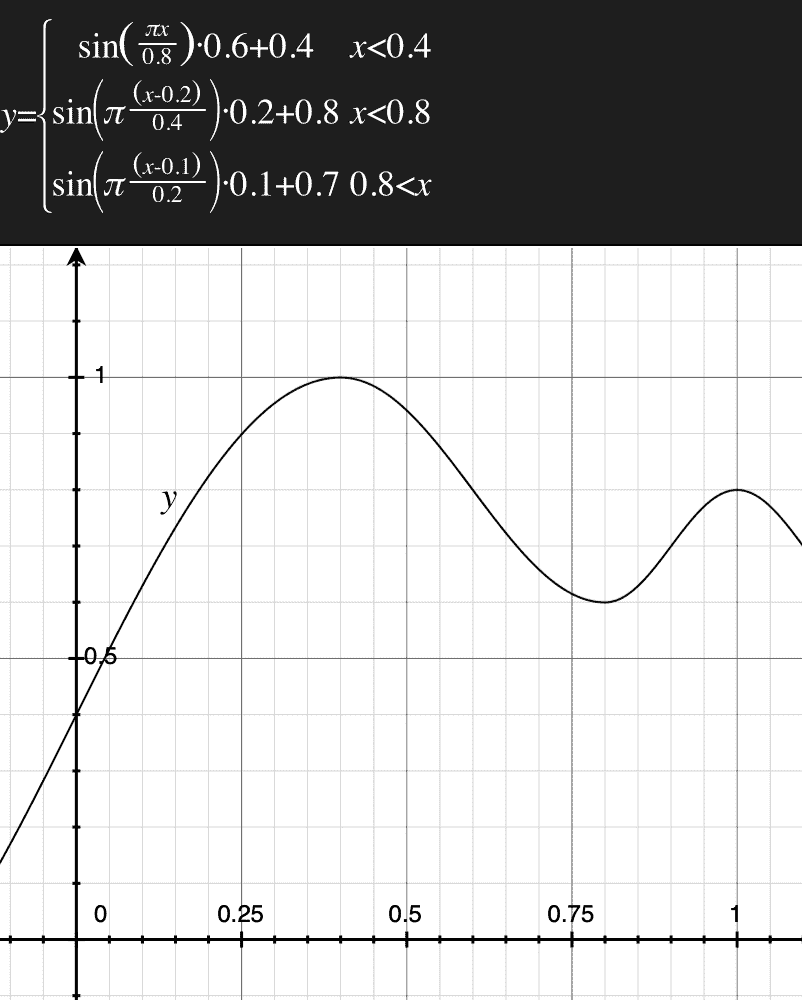Then I used distance transform to turn the outline into “3D”. (Distance transform basically means the distance of each pixel to the closest point on the outline). For many blob-like object (such as a fish), the distance transform can roughly model thickness.

However, using (Euclidean) distance transform, the thickness will grow linearly, which is not too ideal, since fishes usually have more rounded shapes instead of flat facets. So, I eased the z with the equation for a circle to produce a more spherical/cylindrical surface.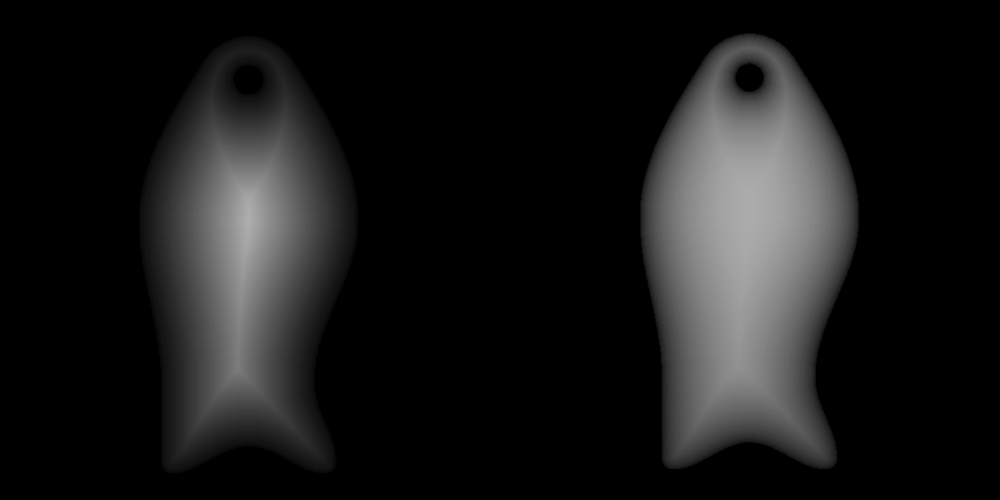It was late on Thursday night so I fell asleep, and dreamt that I modelled the fish shape using another way. In my dream, I rotated the outline along Y-axis and produced a mesh from the trace. I thought it was so much better. But when I woke up I discovered that it didn't make that much sense, since the outline wasn't entirely rotationally symmetric and it would be quite involved to figure out an algorithm that worked. Distance transform was the better (lazier) way; I am smarter when I'm awake.

The next step was to add some fish scales. The pattern itself was pretty trivial to generate, but the hard part is to make one that is machinable. Prof. Neil said that the #1 bane of this week are bubbles; I think bubbles are #2 because I found the fat 1/8in endmill a much more massive headache to design around. The wax is 7 by 3 inches, meaning that with a 0.125 inch endmill, not counting the necessary borders required for mold-making, the “pixel” resolution is only 56x24. That's some pretty low resolution pixel art.

However, I very soon realized that there was a way around this, to make my pattern appear to have much higher resolution. Instead of carving out the pattern itself, I can carve out the negative space around the pattern, so the lines for the pattern (now in relief) can be thinner than 0.125'' limit and thus look more intricate.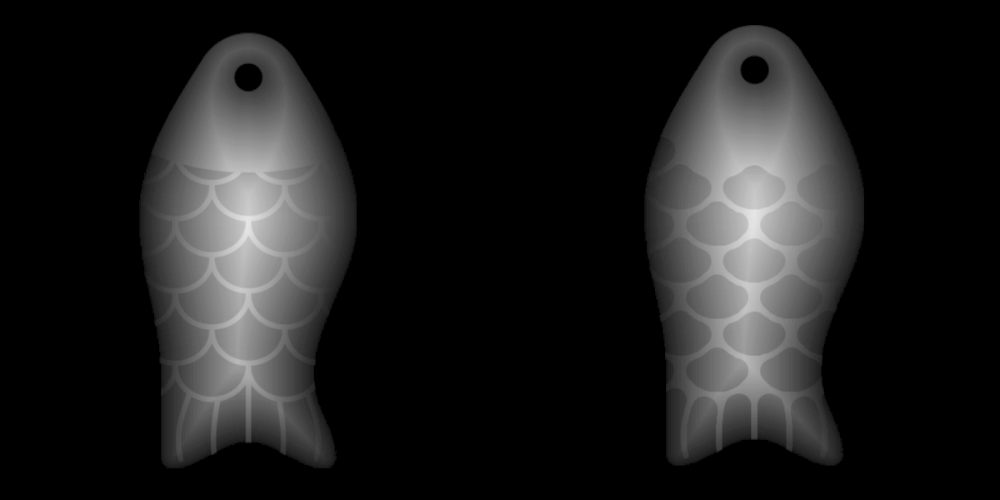However, one problem remained. The cylindrical endmill would still not be able to go in those small corners and articulate detailed shapes (unless the shape is an ellipse). Of course, I could just send the file to the ShopBot and see how much details it ended up milling. But I wanted to get a better idea, or some sort of preview of what the shopbot would be able to do, while I was designing.

I came up with this solution: I first traced each shape that was to be removed by the endmill (negatives) into polygons, then inset these polygons by the radius of the endmill, and finally rendered them with a fat stroke equal to the diameter of the endmill using round line-cap and line-join. This simulates the path of the endmill and I am now able to see what the true result looks like. In some sense, I re-implemented some functionalities of CAM softwares.

Next step was to overlay the fish's scales onto the its body. If I were generating 3D meshes directly, this would have been a pain of moderate severity, for the surface of the fish was curved. Now with depthmaps it's only a matter of blend modes. Initially I imagined an additive blend mode, but it turned out the normal mixing with a gradient mask that further smooths out the sides worked the best.

I also added a small dorsal fin and a tiny pectoral fin. They were painted using “lighter” blend mode (just a max of two layers). (For some reason, every software likes to come up with their own names for the same blend modes, and each of them forget to implement some blend modes. In fact, they couldn't even agree on the name “blend mode”: javascript calls it “global composite operation”).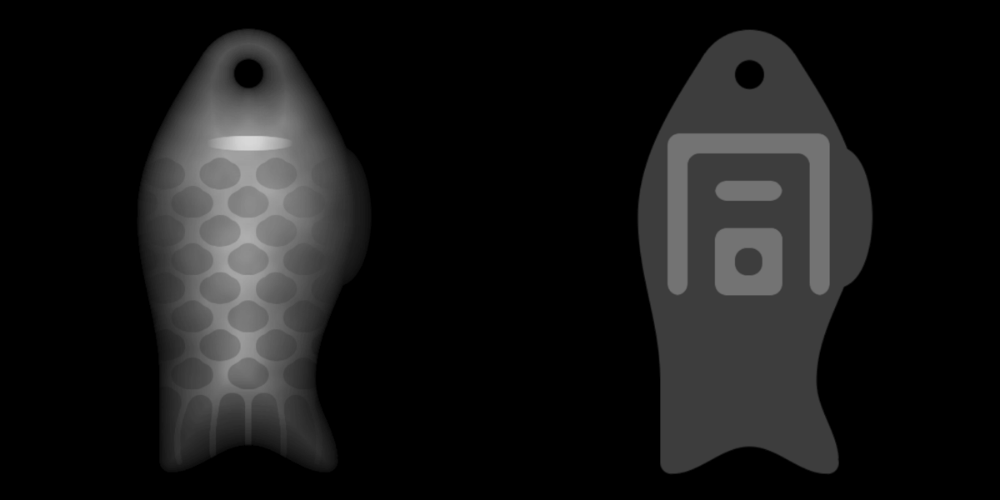Now came the exciting step (but also arguably a simpler one): The positive/negative character on the interior side that would lock into each other. As mentioned, the character is “同”, meaning sameness or equivalence, and pronounced “tóng” in mandarin (or /duŋ/ in middle Chinese, if you prefer to be authentic), and is traditionally the character adorning these kind of tallies.

My initial idea was to draw the character as dot matrix/pixel art, and use bicubic/lanczos interpolation to upsample and threshold, creating smoother turns and corners resembling the style on Chinese seals (old demo I made).

However after some rough layout, I realized that, because the endmill was so fat and the strokes of the character needed to be at least as wide as its diameter, my fish was barely large enough to contain this simple character. Therefore, I would need finer control of the strokes to ensure they're machinable, and couldn't just trust the output of some bicubic upsampler. Because of this, (and that I was too lazy to port my old code), I simply supplied additional information in my dot matrix specifying what rounded shape to draw, a la ASCII art:

 dMMMMMb
M'   "M
M <M> M
M     M
M dMb M
M MOM M
v qMp v


M means a full block, d means a lower-right sector, v means an upper semi-circle, etc.

It seemed to work pretty well. I eroded the negative version by a couple pixels, so that it would be easier to fit them -- It was not a press-fit construction kit, so it did't need to be that tight!

It was Friday and my shop time was not until Saturday morning, so I loaded up my STL model in the shop's Pathworks3D software, to see if it's going to work at all. Seemed good.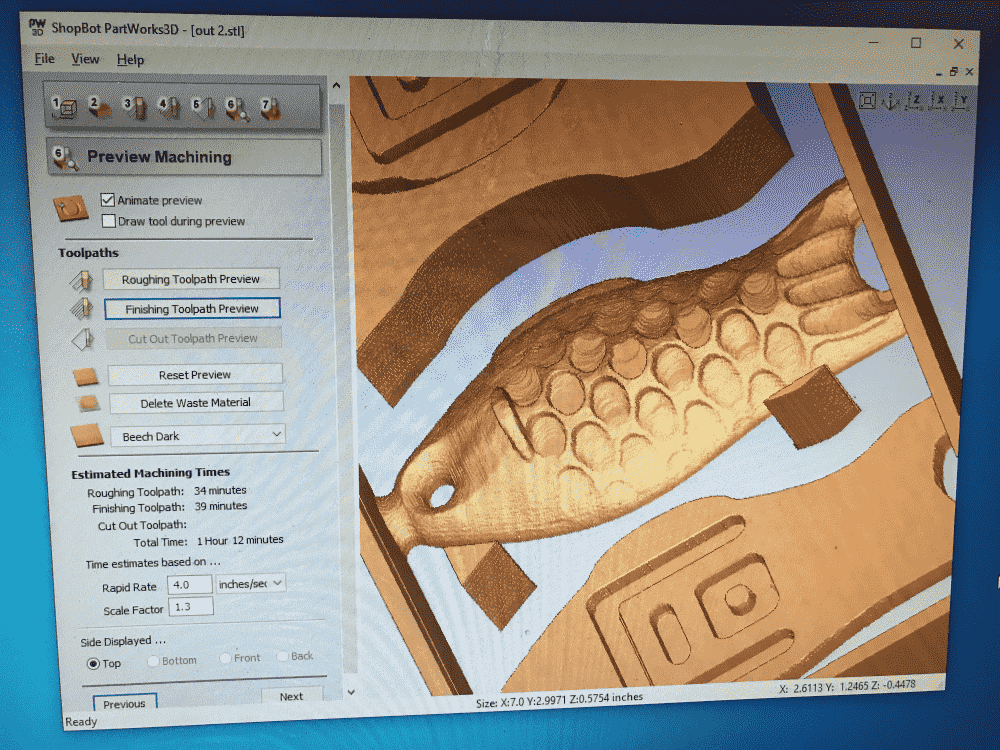I added some walls, registration marks and vents, as required for molding and casting.

Overall I was mostly satisfied with my design, but the greatest itch was its lack of fine details due to limitation of the endmill. I also had to make the fish more chubby than what I had originally imagined in order to fit the character, due to the same reason.

Then I fell asleep again, and had a dream all night where I completely screwed up the machining, in multiple terrible ways I could not recall. So I was very relieved when I woke up next morning, discovering that I got a “second chance” to machine my design.

## Machining

This week we were using a desktop version of the ShopBot we used for CNC week. However, we were told that its smaller size would not stop it from killing us. Therefore I teamed up again with my buddy from last time, Reina, so in case that happened, my corpse could be promptly removed from the shop.

We glue gunned the wax on to a small piece of OSB, which was then screwed onto the bed of the shopbot. I loaded up my STL model into Pathworks3D again, and after a couple minutes of clicking around, managed to export the rough and finishing toolpaths.

However, when we ordered the shopbot to start, it started to mill some thin air not even remotely in the vicinity of my wax block. It was not difficult to realize that the zero in my exported toolpath was wrong.

So I went back to Pathworks3D, and indeed found that the little radio buttons denoting the origin needed to be clicked.

However, when we ordered the shopbot to start again, it again started to mill some far-away air. Then I realized that I forgot to click “Apply” after chaning the origin.

The third time it finally worked. Reina shot some timelapses of the machine at work while I was busy vacuuming the shavings:

The rough toolpath took some 10-20 minutes to mill, while the finishing toolpath took somewhat longer; Overall it probably took a bit less than an hour. The machined wax looked quite nice. (It would probably look even nicer if it was not this obnoxious shade of blue; If they didn't know what a pretty color is, why couldn't they just make it gray or something?)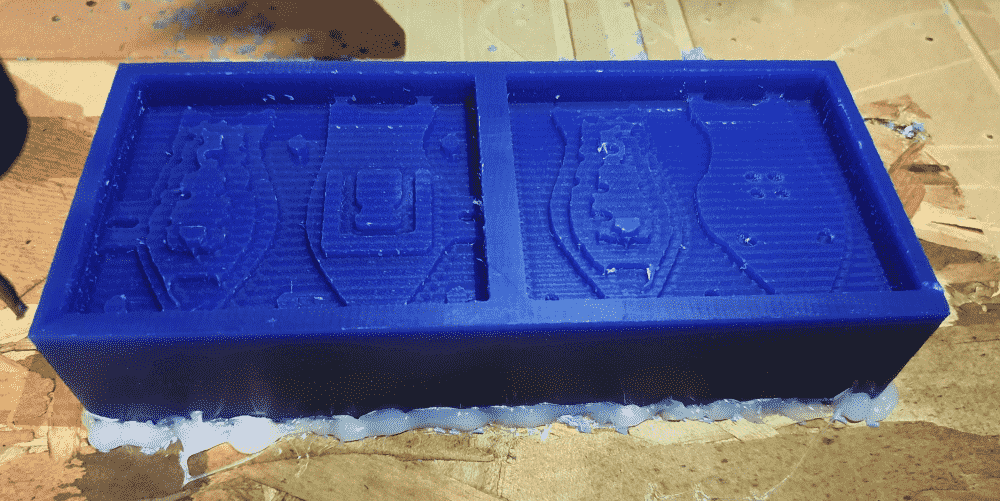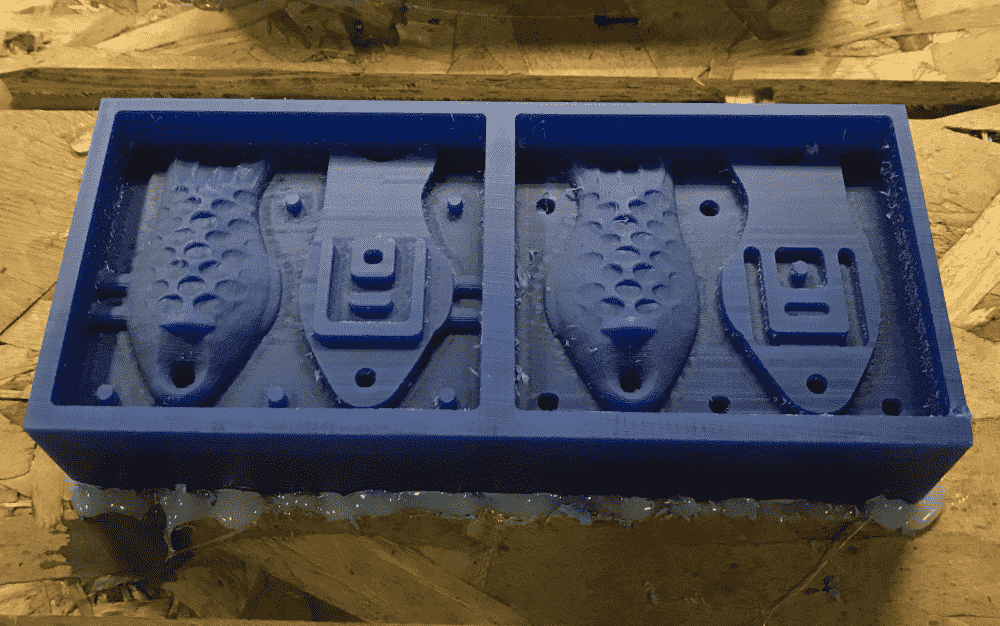## Molding

After machining the wax, we went on to create the molds from OOMOO. OOMOO comes in two parts, one is a bluish liquid and one is a greenish liquid. We stirred the two bottles thoroughly for 3 minutes, and mixed them together for another 3 minutes. They were sticky liquids and somewhat hard to stir, but using superman arm muscles I grew two weeks ago from hammering OSB boards all day long, I stirred them relatively painlessly.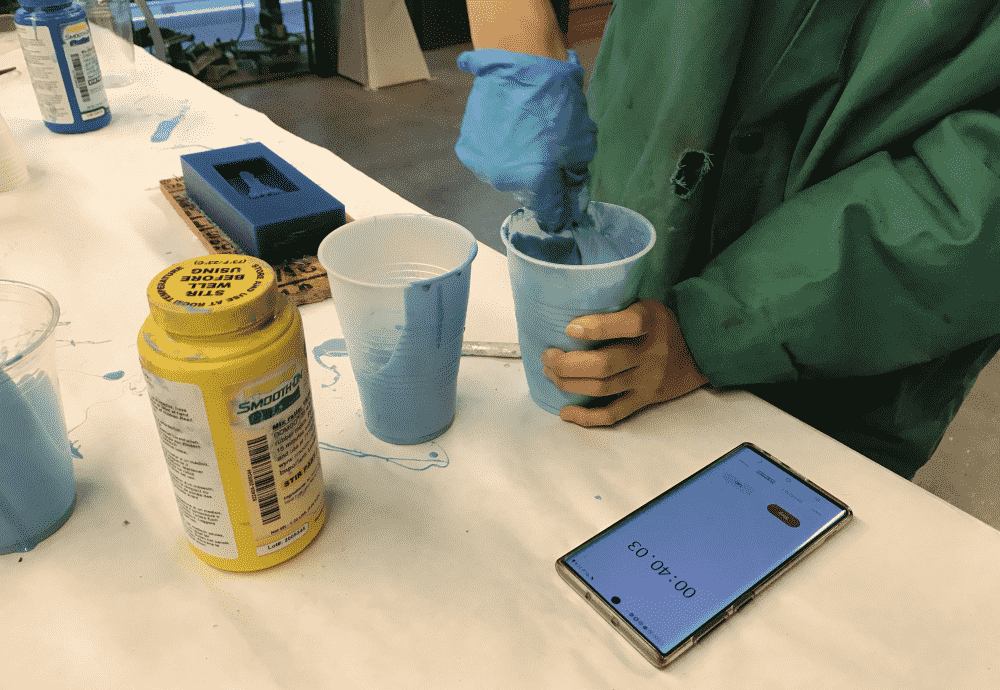Next, I poured the OOMOO into my mold. I tried to pour it in a thin stream to reduce the bubbles; However it was questionable how well I performed it, since there were still quite a few bubbles. Then we did a bunch of other stuff since OOMOO takes a long time to dry.

## Casting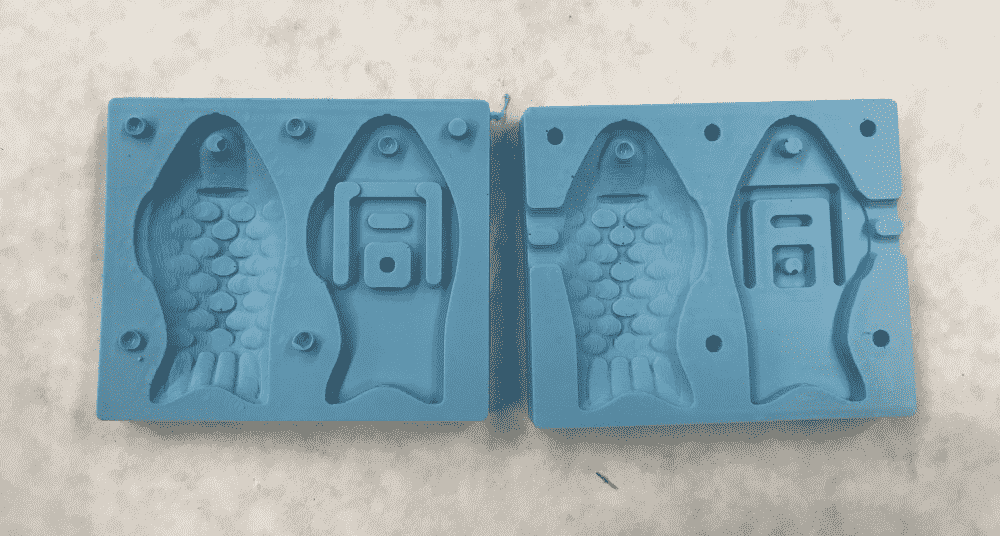When the OOMOO appeared dry enough I peeled it off the wax. They turned out nice and clean, save for some small bubbles. The registration marks turned out terrible though; each of them had a huge bubble in it. Moreover, they had the reverse effect of making it harder, instead of easier, to align the molds due to their rubbery nature and tendency to bounce off each other instead of fitting into each other.

Naturally I wanted to make my cast in metal. We put a block of cerrotru, a brittle alloy, into a tiny pot to cook it. It was a quite poetic sight, watching the metal melt like butter into a silvery stew.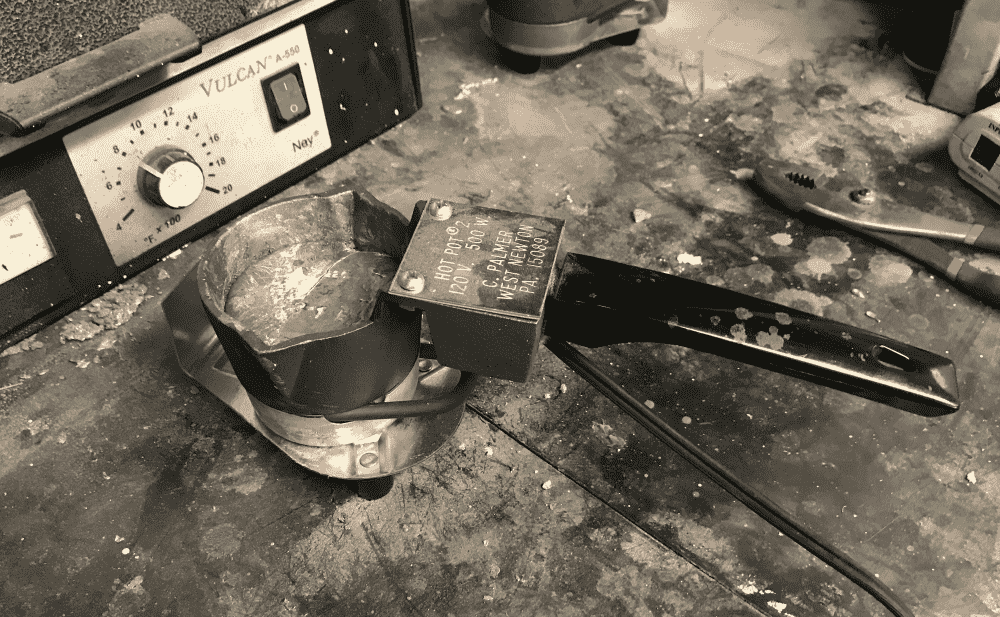After dusting the molds with baby powder, we tried to tape them together, so they can work as a 2-part mold as intended. However, neither masking tape nor duck tape liked OOMOO. They simply lost all stickiness when being applied to it. In the end we squeezed the molds together with two heavy boxes (which coincidentally contained more bottles of OOMOO).

Since I did not trust my shaky hands, I held the boxes in place while Reina helped pour in the metal. It seemed that the metal refused to get into the hole, and instead happily flowed all over the place. We literally made a hot mess. Refusing to admit defeat, I asked her to pour even more; We ended up with a even more giant hot mess.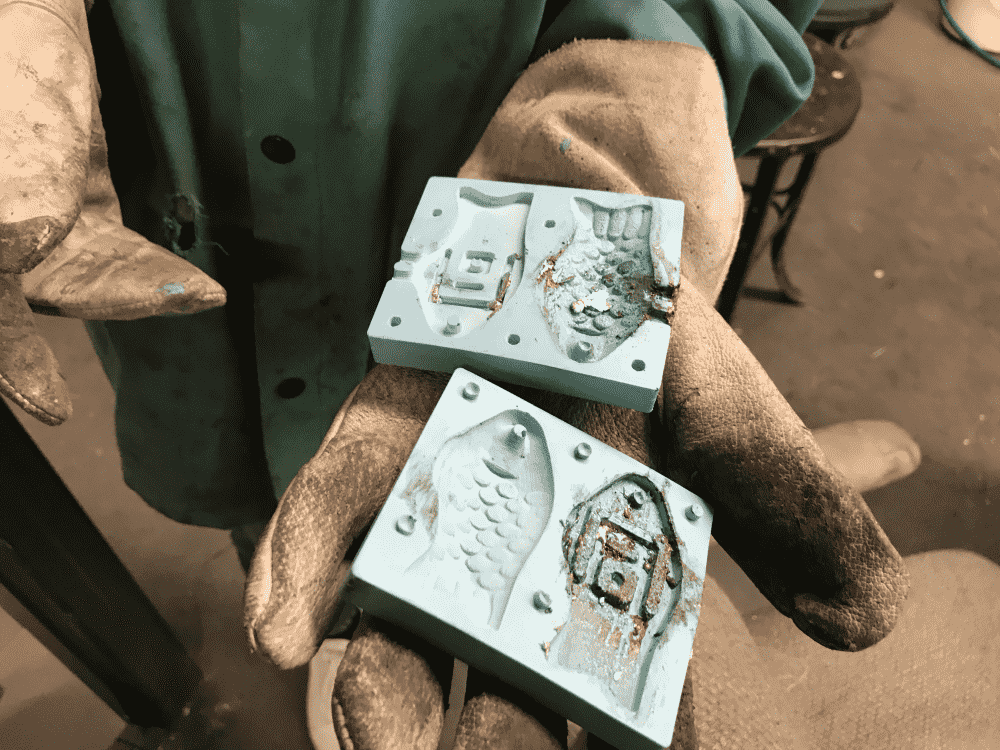The OOMOO mold was so burnt and warped by the hot metal. Gross! We tried to wash it but it looked like it was permanently damaged.

The good thing was I still had my wax block. We immediately started off making a new OOMOO mold from it. Meanwhile, I wanted to try out the damaged mold as a test: I was curious to see how bad it can get. Instead of using it as a 2-part mold, we poured metal into each of the 4 shapes.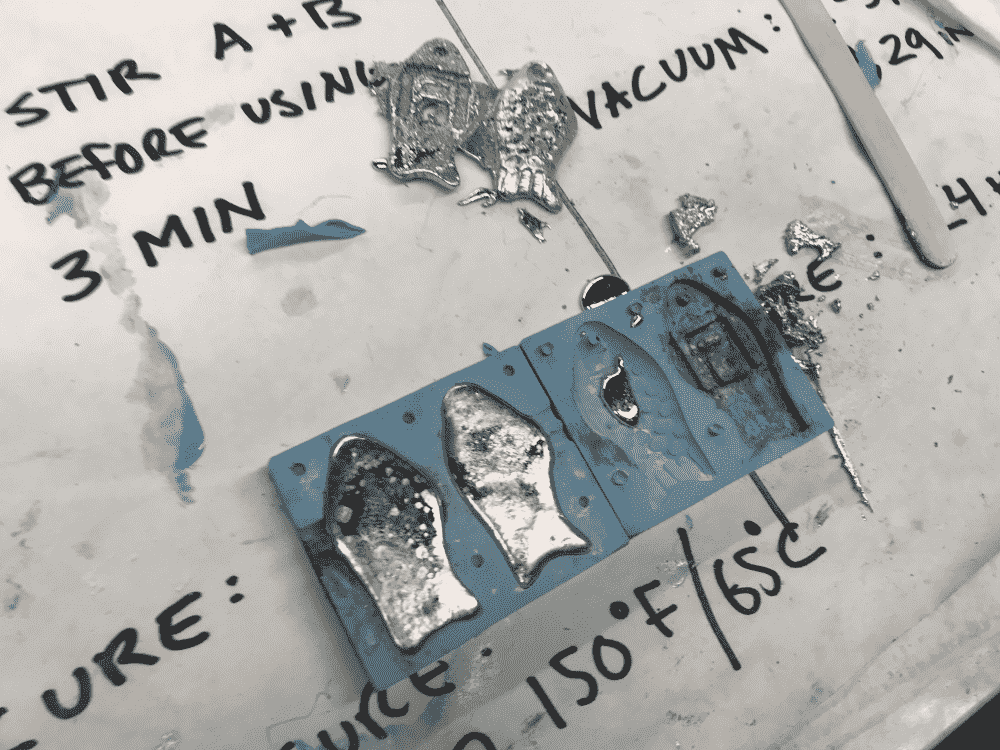It went out not bad! The burning and warping definately had detrimental effects, but I did get roughly the shapes I had designed.

The positive/negative characters did not fit each other perfectly; Yet they still fit partially: if they were stacked on the table and the top piece was moved in any direction, the bottom piece would be dragged along; so they did kind of lock into each other.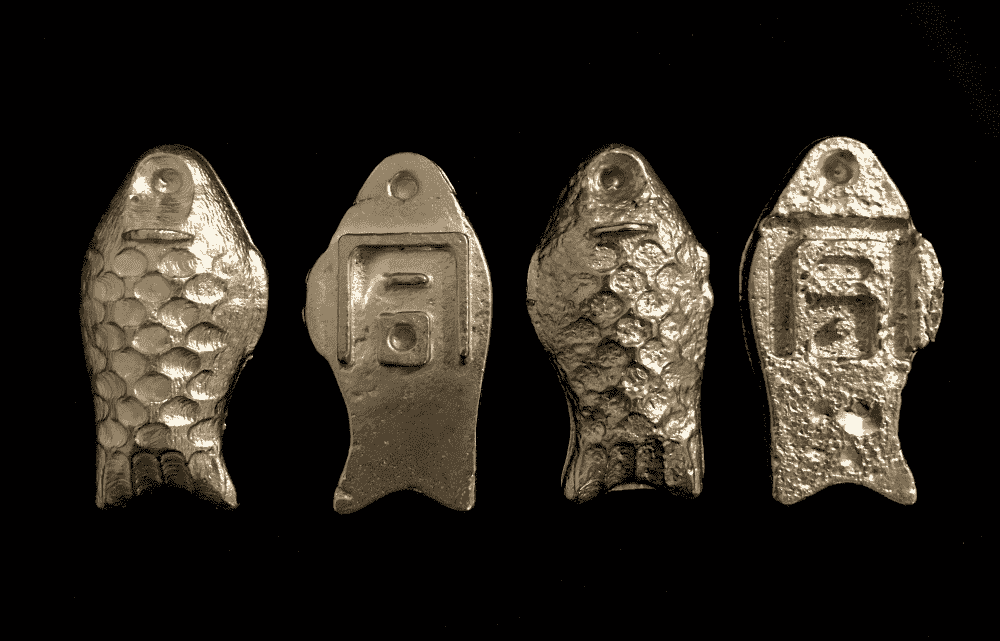We decided to call it a day and meet again on Monday, with our TA Graham to find out what to do next. We figured that we would either use some epoxy glue to glue the 4 pieces into 2, or properly learn how to make the 2-part mold work.

## How Graham's Amazing Tricks Made It Work

In an email Graham explained that I should make my holes much larger, and add a vent near the fish tail (because as a local maximum it might trap air). Then I could align and fix the molds by nailing together a wooden box around them.

However, when we came in on Monday, Graham showed us some cool stuffs that rendered the wooden box idea obsolete. They were 4 pieces of L-shaped wood, and when clamped together with clamps, could fit and squeeze anything with a rectangular cross section. What an ingenious design!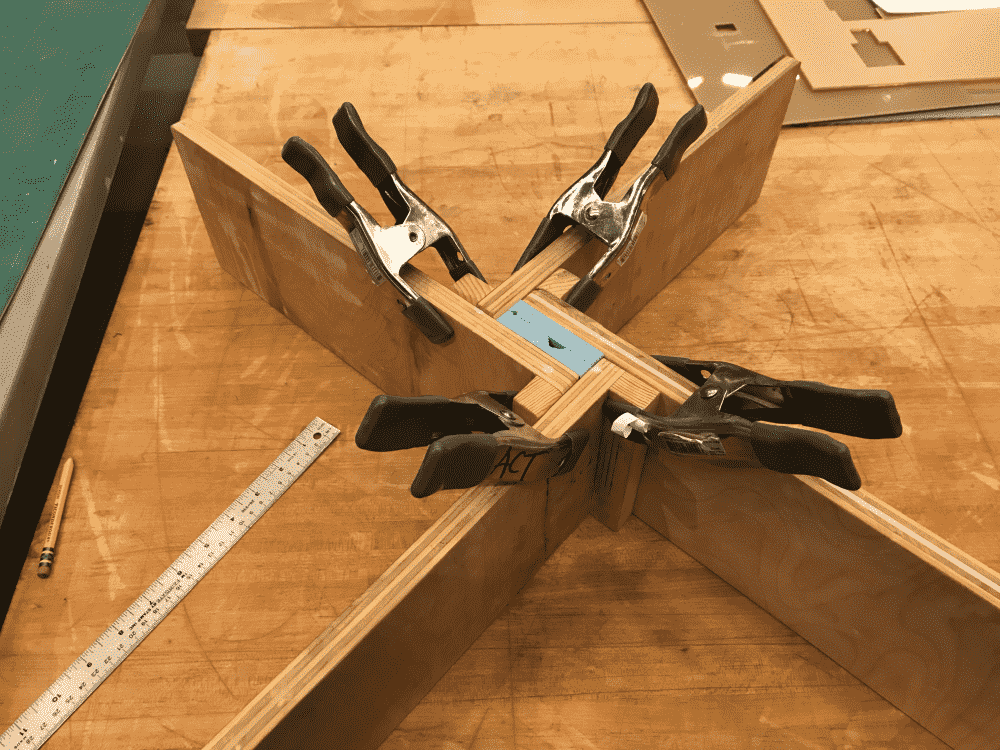After cutting the holes and vents as Graham suggested, and chopping off the registration marks as they were more annoying than they were helpful, we clamped the L pieces around the molds. Spotting a nearby stool, Graham flipped it upside-down and rested the frame on its beams, making it possible to add clamps to both the top and the bottom of the frame, to further sturdy it.

This time with the enlarged holes, I managed to pour the metal into the mold relatively painlessly. Graham pointed out that the mold was fully filled, since metal was starting to come out from the vent. I thought it was a nice indicator, otherwise I would have no way of knowing.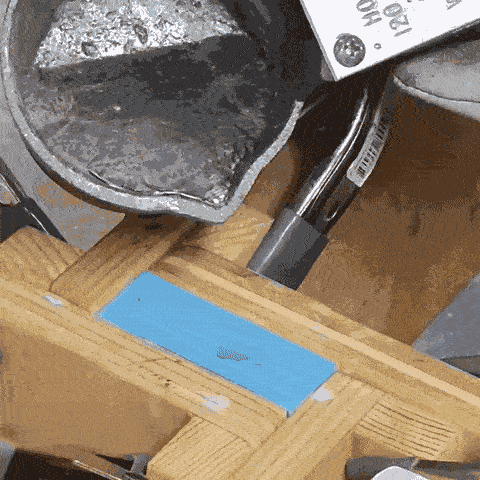Then Graham decided to jiggle the molds a bit. All of a sudden, the hot metal was discharged from the bottom of the mold, like a spectacular diarrhoea. All it gulped moments ago was pooped out, and became a hot mess on the floor. What a sight.

Graham inferred that it was probably the inner wall between the two fish shapes becoming leaky due to the jiggling. He said the molds were to be cleaned, and the pouring to be performed again, but this time sans the jiggling: “Don't poke the bear!”

So we tried again, this time with even more clamps, and remembered to not poke the bear. After a couple minutes, the cast came out perfect. “You can throw away your old casts now” said Reina. “What a waste of \$9 on epoxy metal glue I bought yesterday” I thought.

The cast had some extra artifacts from the hole and the vent, and Reina helped saw those off. However, not knowing that my fish had a dorsal fin, she sawed the fin off too. Oops!

The middle hole in the 口 of 同 character was gummed because there was a bubble in the OOMOO mold, so we tried to drill it. We used a power tool as suggested by Graham, but since the surface was bulging out, the power tool slipped around it. So we first hammered a dent onto the bulge, and then drilled it. That worked. The only endmill with the right diameter was V-shaped, so the hole became conic.

Meanwhile, I casted the other half of the fish using the same procedure as before. It also came out well; However there was still a problem: the two pieces still did not fit perfectly. They did lock onto each other like my old casts, but when pressed together, they leave a large seam in the middle and the fit was crooked.

Observing the pieces carefully I thought I spotted the problem: though I designed the width of the strokes to be 1/100 inch wider than the endmill diameter (so that the endmill should be able to fit all the way in), probably due to some anti-aliasing and quantization misfortunes, some strokes ended up slightly deeper than others when Pathworks3D computed the toolpath. Ahhh!!!

One way was to sand down the positive piece, as this would be easier than drilling the negative piece. But I insisted that it would also make it less cool. So Graham introduced me to a machine that has a clamp on the bottom and drill thing on the top. There're two screws to adjust X/Y position, and a crank to lower the drill. We tried to deepen the shallow grooves on the negative piece with that. It did something, but apparently not enough: the pieces still didn't fit perfectly.

So Graham had to show me a more awesome tool, located upstairs. It looks like a fat pen, whose tip goes spinning really fast. I could hold the pen with my bare hands, and stab at things I wanted to do away with. A foot pedal controls the speed. Initially, I was clumsy and the pen wanted to fly away. But soon I learned the knack and decided that I liked the tool. After a couple iterations the grooves became deep enough, and the fit was finally perfect. I also re-drilled the hole of the 口 to make the conic hole cylindrical. Nice!

Overall I was quite happy with how my cast turned out, even though it had some minor scars and defects due to various accidents along the way. I definitely expected challenges when I decided to make a two-part mold of two parts which then need to fit together. But like all previous weeks, thanks to help from TA's and classmates, I managed to pull it off. Yay!